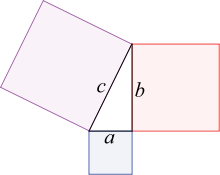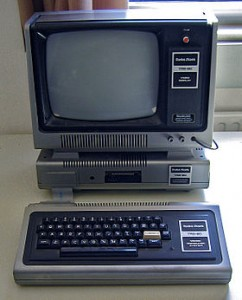# Pythagorean Triples -- Simple Yet Fascinating

May 25 2011

Many of us would have learned about the Pythagoras’ Theorem during Maths class in our secondary school days. Yup, it’s that famous a2 + b2 = c2 equation that relates to the sides of a right-angled triangle.Pythagoras' Theorem, depicted with an upright triangle. The angle formed by the legs a and b is a right angle (90o). All such triangles have the property of a2 + b2 = c2

The diagram on the right illustrates Pythagoras’ Theorem using an upright triangle. This is probably the view most students are familiar with. However, the view according to Euclid, the “Father of Geometry, has the triangle sitting on its hypothenuse, i.e. the longest side, c.

Indeed, an ancient Babylonian tablet (the YBC 7289) which has inscriptions of a triangle for computing square root of 2 (a special case of Pythagoras’ where a = b = 1) showed the same triangle orientation. This suggests the right-angled triangle property was known way before Pythagoras, as mentioned in books such as “The Pythagorean Theorem — A 4000 Year History“.

For a triangle with sides of integer lengths, the smallest possible values of a, b and c (assuming a < b) are a=3, b=4 and c=5; indeed, 32 + 42 = 52. This is a Pythagorean triple, commonly written as (3, 4, 5). The next triple is (5, 12, 13), followed by (7, 24, 25).

A primitive Pythagorean triple occurs when a, b and c are co-prime (or relatively prime), i.e. they do not have any common positive divisor other than 1. Thus, while both (3, 4, 5) and (6, 8, 10) are triples, the former is primitive while the latter isn’t.

To find Pythagorean triples, we could use a brute force method of going through successive integers, something suited for a computer to crunch (see footnote). Or we can use the clever algorithm formalized in “Euclid’s Elements” in this manner:

1. Choose 2 integers u and v with these criteria:
i) u > v
ii) u relatively prime to v
iii) one odd and the other even
2. Compute a = 2uv
3. Compute b = u2 – v2
4. Compute c = u2 + v2
5. We get a primitive Pythagorean triple in the form (a, b, c) or (b, a, c)

Examples:

• With u=2 and v=1, we get a=4, b=3 and c=5, or the triple (3, 4, 5)
• With u=5 and v=2, we get a=20, b=21 and c=29, or the triple (20, 21, 29)
Here is a list of primitive Pythagorean triples with values less than 100:

 (3, 4, 5) (5, 12, 13) (7, 24, 25) (8, 15, 17) (9, 40, 41) (11, 60, 61) (12, 35, 37) (13, 84, 85) (16, 63, 65) (20, 21, 29) (28, 45, 53) (33, 56, 65) (36, 77, 85) (39, 80, 89) (48, 55, 73) (65, 72, 97)

Maths can be a very dry and boring subject, yet when we look at its peculiarities, we can have lots of WittyCulus fun with numbers, don’t you agree? Think positive (Math pun?), try to appreciate all those equations, formulae, theorems, etc you’ve learned and who knows, you may define (oops, another one!) your world from another angle!

———-

#### Looking Back…I first dabbled with computers when I was in Sec 2. In those good old days, the object of desire was the Tandy Corp’s Radio Shack TRS-80 microcomputer (see pix on left) that ran on a Z80 CPU (central processing unit — the computer’s “brains”).

To program the TRS-80, I learned the BASIC programming language. TRS-80 came with the Level II BASIC interpreter in ROM, code that was licensed from the then-young Microsoft company. Incidentally, Bill Gates had worked on enhancing this BASIC interpreter firmware code himself.

Yes, that was the “blue screen” software giant in its infancy…

My fascination with Maths and how computers can do things real quick soon saw me writing a BASIC program to find Pythagorean triples. This was a really simple three-loop routine that ran through each successive larger number and computed squares for comparison; when a match occurred, that triple was printed out.

The BASIC code went something like the following. This is as much as I can recall, but may not be in the original “flavour” of Level II BASIC.

```10 FOR A=3 TO 100 20 FOR B=A+1 TO 100 30 C=B+1 40 IF (A*A + B*B) = C*C THEN PRINT A, B, C : GOTO 70 50 IF (A*A + B*B) > C*C THEN C=C+1: GOTO 40 60 NEXT B 70 NEXT A ```

I didn’t own a TRS-80 until a while later, so I was hanging out with some computer “nuts” at the OG department store in People’s Park Complex. Betcha didn’t know that OG sold computers! I mean, they sold ladies clothes (that our young and/or hip would rather die than being seen in them; sorry :-)) and shoes, and had a nice in-house restaurant on the third floor… But I digressed.

While playing with the Pythagorean triples program one day, I had the good fortune of meeting an experienced programmer named John (I believe he was a Fortran programmer) who showed me how arrays and lookup tables could speed my search for triples tremendously. I was quickly won over and that was one reason why a young programmer went even deeper into how computers work.

As they say, the rest is history. But such is the stuff that makes life interesting — it sure does bring back fond memories (including how we used to tease the OG staff, people like Rita, Lillian, Jennifer, Doris ;-))